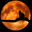Related Tags

python
pandas
values_counts
data analysis
community creator

# How to count unique items in pandasNeko Yan

pandas provides the useful function value_counts() to count unique items – it returns a Series with the counts of unique values.

## Category data value count

First of all, let’s see a simple example without any parameter:

import pandas as pd
import numpy as np

# create a dataframe with one column
df = pd.DataFrame({"col1": ["a", "b", "a", "c", "a", "a", "a", "c"]})
# print the dataframe object
print(df)
# line break
print("=" * 30)
# counting unique items
item_counts = df["col1"].value_counts()
print(item_counts)
count unique items
• From the output of line 10 you can see the result, which is a count of the column col1.

## Category data value count with normalize

Sometimes, we don’t care about the exact number for each item of one column, but we care about the relative percentage. Setting normalize=True can return the percentage instead of the counts.

import pandas as pd

# create a dataframe with one column
df = pd.DataFrame({"col1": ["a", "b", "a", "c", "a", "a", "a", "c"]})

# setting normalize=True
item_counts = df["col1"].value_counts(normalize=True)
print(item_counts)
count unique items with normalize
• line 7 sets normalize=True.

• From the output of line 8, you can see the difference from the last demo: the output is a relative percentage, not counts.

## Continuous data bucket intervals

value_counts() can handle continuous values as well as category values. By setting bin=n parameters, you can group those continuous values into n groups.

import pandas as pd
import numpy as np

# create a array with random value between 0 and 1
data = np.random.random((30,))
# create a DataFrame object from array
df = pd.DataFrame(data, columns=["col1"])
# show the first five rows of this dataframe object
# line break
print("=" * 30)
# set bins=8
value_bins = df['col1'].value_counts(bins=8)
print(value_bins)
Continuous data bucket intervals
• line 12 sets bins=8.

• From the output of line 13, you can see that the original values are grouped into 8 bins.

RELATED TAGS

python
pandas
values_counts
data analysis
community creator

CONTRIBUTORNeko Yan
RELATED COURSES

View all Courses

Keep Exploring

Learn in-demand tech skills in half the time